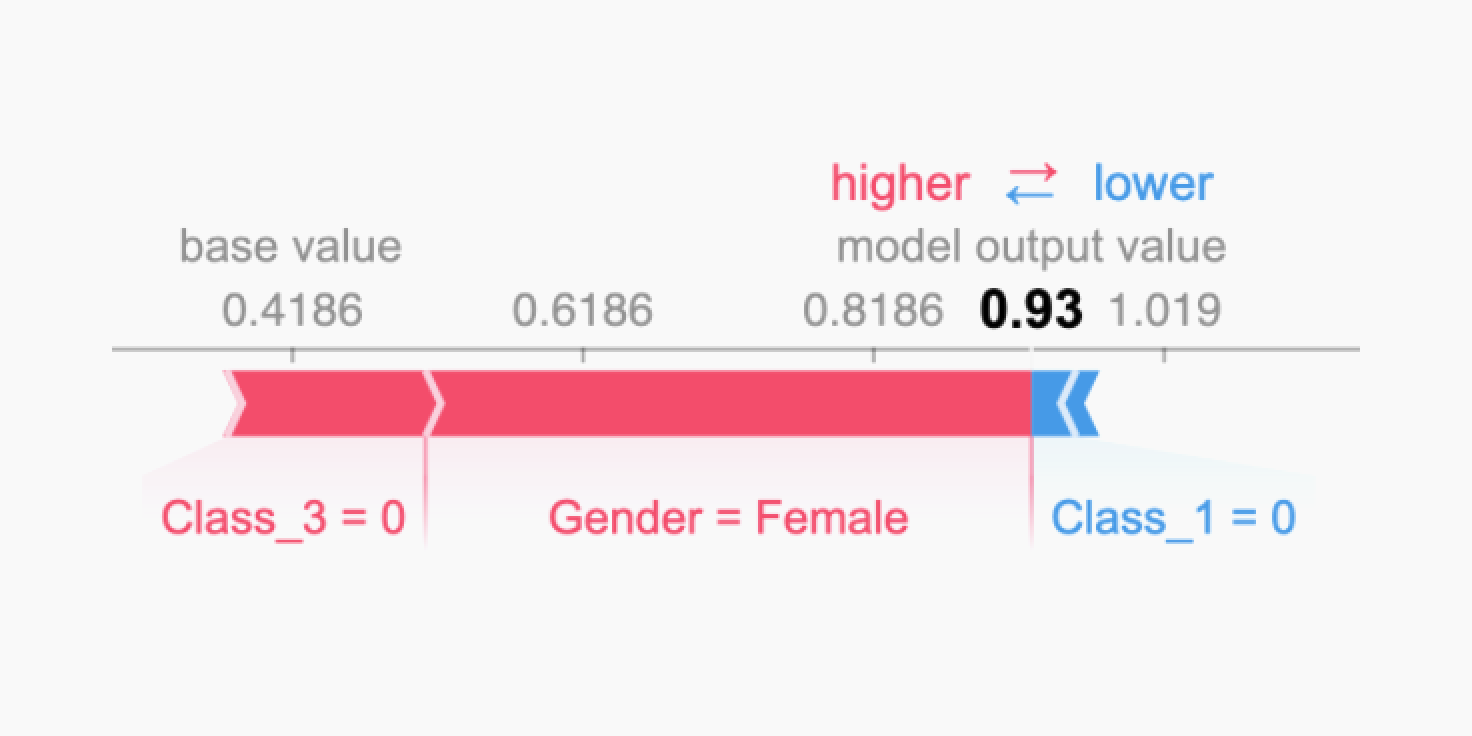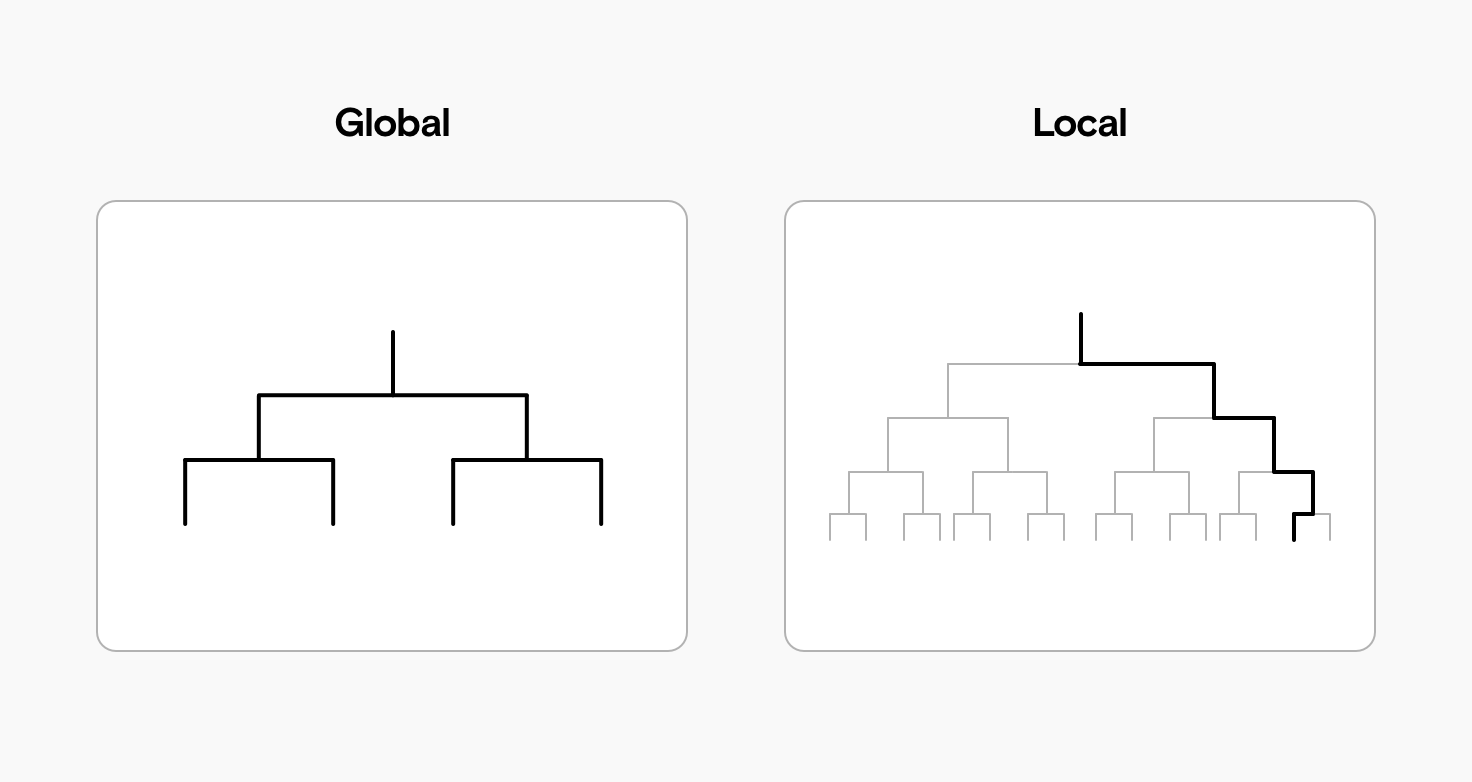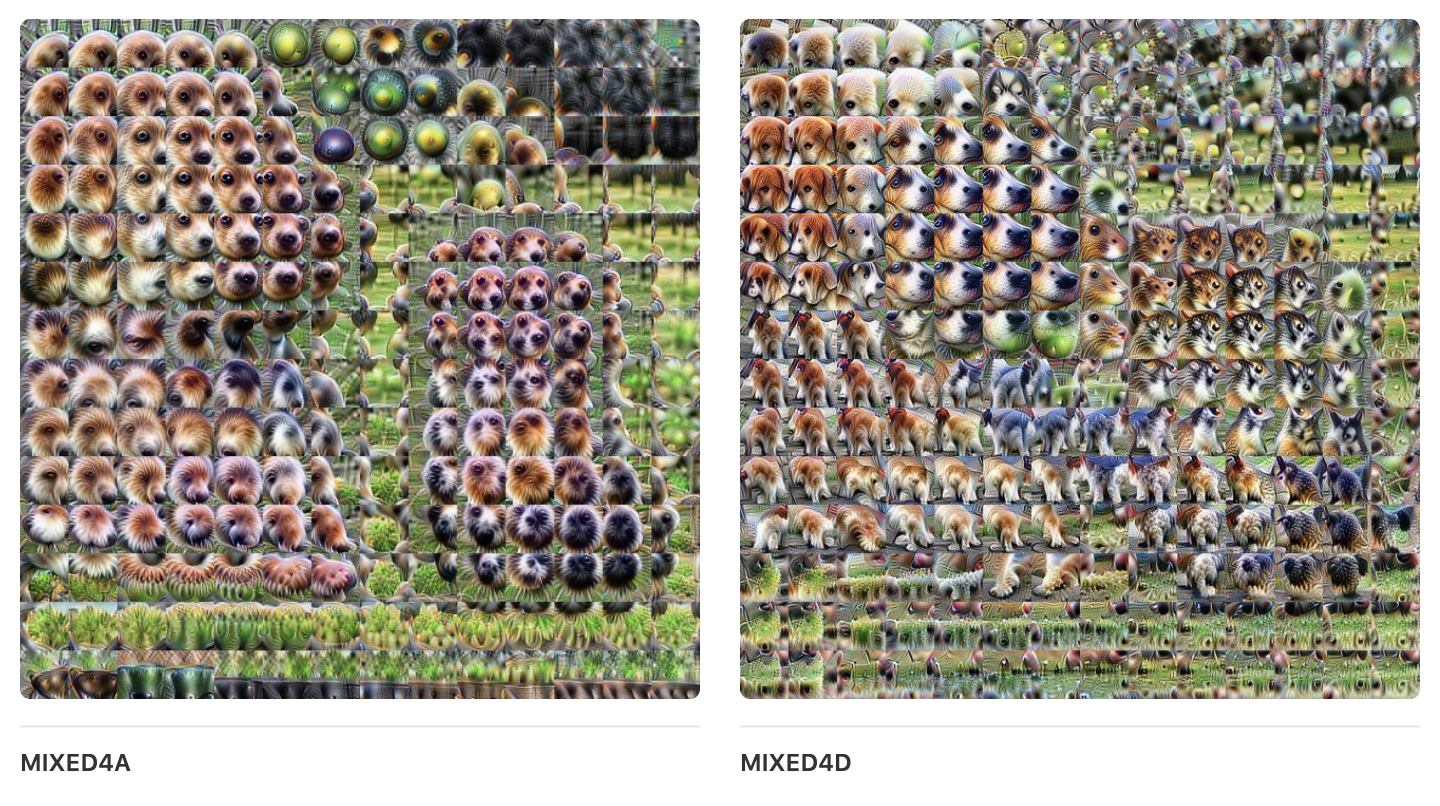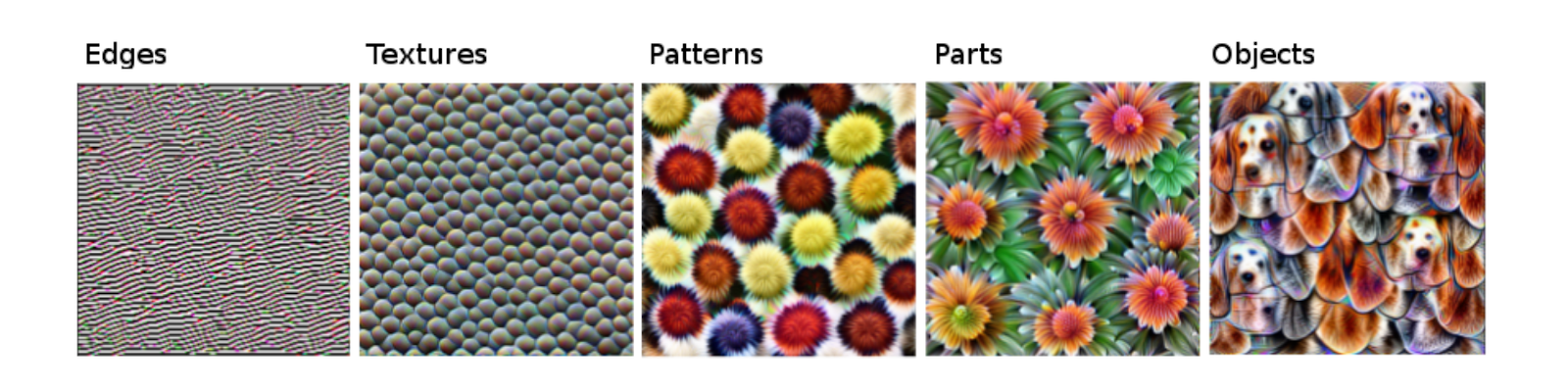Oops! Something went wrong while submitting the form.

# Interpretable Machine Learning

Why and how to make your machine learning models interpretableby
Markus Schmitt
Markus Schmitt

Critics of machine learning say it creates “black box” models: systems that can produce valuable output, but which humans might not understand.

That’s a misconception. Machine learning can be interpretable, and this means we can build models that humans understand and trust. Carefully constructed machine learning models can be verifiable and understandable. That’s why we can use them in highly regulated areas like medicine and finance.

## What is an interpretable model?

When humans easily understand the decisions a machine learning model makes, we have an “interpretable model”. In short, we want to know what caused a specific decision. If we can tell how a model came to a decision, then that model is interpretable.

For example, we can train a random forest machine learning model to predict whether a specific passenger survived the sinking of the Titanic in 1912. The model uses all the  passenger’s attributes – such as their ticket class, gender, and age – to predict whether they survived.

Now let’s say our random forest model predicts a 93% chance of survival for a particular passenger. How did it come to this conclusion?

Random forest models can easily consist of hundreds or thousands of “trees.” This makes it nearly impossible to grasp their reasoning.

But, we can make each individual decision interpretable using an approach borrowed from game theory.

SHAP plots show how the model used each passenger attribute and arrived at a prediction of 93% (or 0.93). In the Shapely plot below, we can see the most important attributes the model factored in.

• the passenger was not in third class: survival chances increase substantially;
• the passenger was female: survival chances increase even more;
• the passenger was not in first class: survival chances fall slightly.Gender and class are the most important features in a model predicting survival chances of Titanic passengers.

We can see that the model is performing as expected by combining this interpretation with what we know from history: passengers with 1st or 2nd class tickets were prioritized for lifeboats, and women and children abandoned ship before men.

By contrast, many other machine learning models are not currently possible to interpret. As machine learning is increasingly used in medicine and law, understanding why a model makes a specific decision is important.

## What do we gain from interpretable machine learning?

Interpretable models help us reach lots of the common goals for machine learning projects:

• Fairness: if we ensure our predictions are unbiased, we prevent discrimination against under-represented groups.
• Robustness: we need to be confident the model works in every setting, and that small changes in input don’t cause large or unexpected changes in output.
• Privacy: if we understand the information a model uses, we can stop it from accessing sensitive information.
• Causality: we need to know the model only considers causal relationships and doesn’t pick up false correlations;
• Trust: if people understand how our model reaches its decisions, it’s easier for them to trust it.

## Are some algorithms more interpretable than others?

Simpler algorithms like regression and decision trees are usually more interpretable than complex models like neural networks. Having said that, lots of factors affect a model’s interpretability, so it’s difficult to generalize.

With very large datasets, more complex algorithms often prove more accurate, so there can be a trade-off between interpretability and accuracy.

## Scope of interpretability

By looking at scope, we have another way to compare models’ interpretability. We can ask if a model is globally or locally interpretable:

• global interpretability is understanding how the complete model works;
• local interpretability is understanding how a single decision was reached.A model is globally interpretable if it’s small and simple enough for a human to understand it entirely. A model is locally interpretable if a human can trace back a single decision and understand how the model reached that decision.

A model is globally interpretable if we understand each and every rule it factors in. For example, a simple model helping banks decide on home loan approvals might consider:

• the applicant’s monthly salary,
• the size of the deposit, and
• the applicant’s credit rating.

A human could easily evaluate the same data and reach the same conclusion, but a fully transparent and globally interpretable model can save time.

In contrast, a far more complicated model could consider thousands of factors, like where the applicant lives and where they grew up, their family’s debt history, and their daily shopping habits. It might be possible to figure out why a single home loan was denied, if the model made a questionable decision. But because of the model’s complexity, we won’t fully understand how it comes to decisions in general. This is a locally interpretable model.

## Interpretability vs. explainability for machine learning models

The global ML community uses “explainability” and “interpretability” interchangeably, and there is no consensus on how to define either term.

That said, we can think of explainability as meeting a lower bar of understanding than interpretability.

A machine learning model is interpretable if we can fundamentally understand how it arrived at a specific decision.

A model is explainable if we can understand how a specific node in a complex model technically influences the output.

If every component of a model is explainable and we can keep track of each explanation simultaneously, then the model is interpretable.

Think about a self-driving car system. We might be able to explain some of the factors that make up its decisions. The image below shows how an object-detection system can recognize objects with different confidence intervals.

This model is at least partially explainable, because we understand some of its inner workings. But it might still be not possible to interpret: with only this explanation, we can’t understand why the car decided to accelerate or stop.

## Model-agnostic interpretation

So we know that some machine learning algorithms are more interpretable than others. But there are also techniques to help us interpret a system irrespective of the algorithm it uses.

For example, earlier we looked at a SHAP plot. This technique works for many models, interpreting decisions by considering how much each feature contributes to them (local interpretation).

We can use other methods in a similar way, such as:

• Partial Dependence Plots (PDP),
• Accumulated Local Effects (ALE), and
• Local Surrogate (LIME).

These algorithms all help us interpret existing machine learning models, but learning to use them takes some time.

## “Building blocks” for better interpretability

Models like Convolutional Neural Networks (CNNs) are built up of distinct layers. When used for image recognition, each layer typically learns a specific feature, with higher layers learning more complicated features.

We can compare concepts learned by the network with human concepts: for example, higher layers might learn more complex features (like “nose”) based on simpler features (like “line”) learned by lower layers.

We can visualize each of these features to understand what the network is “seeing,” although it’s still difficult to compare how a network “understands” an image with human understanding.Image recognition CNNs build up conceptual “building blocks” so we can better interpret how the model functions as a whole (Source: Olah et al).

## A hierarchy of featuresDifferent layers of a CNN recognize simpler or more complex features, from simple edge detection to full objects (Source: Molnar: Interpretable Machine Learning).

We can draw out an approximate hierarchy from simple to complex. To interpret complete objects, a CNN first needs to learn how to recognize:

• edges,
• textures,
• patterns, and
• parts.

Each layer uses the accumulated learning of the layer beneath it. So the (fully connected) top layer uses all the learned concepts to make a final classification.

We can look at how networks build up chunks into hierarchies in a similar way to humans, but there will never be a complete like-for-like comparison. Looking at the building blocks of machine learning models to improve model interpretability remains an open research area.

## Example-based explanations

In the SHAP plot above, we examined our model by looking at its features. By comparing feature importance, we saw that the model used age and gender to make its classification in a specific prediction.

A different way to interpret models is by looking at specific instances in the dataset. The distinction here can be simplified by honing in on specific rows in our dataset (example-based interpretation) vs. specific columns (feature-based interpretation).

We should look at specific instances because looking at features won’t explain unpredictable behaviour or failures, even though features help us understand what a model cares about.

### Counter-factual explanations

We can gain insight into how a model works by giving it modified or counter-factual inputs. Knowing the prediction a model makes for a specific instance, we can make small changes to see what influences the model to change its prediction.

In our Titanic example, we could take the age of a passenger the model predicted would survive, and slowly modify it until the model’s prediction changed.

By “controlling” the model’s predictions and understanding how to change the inputs to get different outputs, we can better interpret how the model works as a whole – and better understand its pitfalls.

## Debugging and auditing interpretable models

Machine learning models can only be debugged and audited if they can be interpreted. If a model gets a prediction wrong, we need to figure out how and why that happened so we can fix the system.

Imagine we had a model that looked at pictures of animals and classified them as “dogs” or “wolves.” It seems to work well, but then misclassifies several huskies as wolves.

If we can interpret the model, we might learn this was due to snow: the model has learned that pictures of wolves usually have snow in the background. This works well in training, but fails in real-world cases as huskies also appear in snow settings.

## Does your company need interpretable machine learning?

We love building machine learning solutions that can be interpreted and verified. Reach out to us if you want to talk about interpretable machine learning.